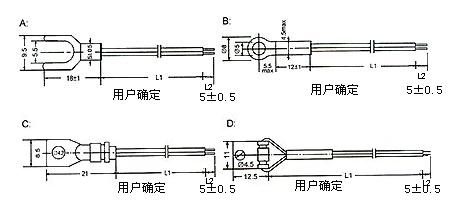﻿ Sensor linearity_

China sensor manufacturers

# Sensor linearity

usually, The actual static characteristic output of the sensor is a bar curve rather than a straight line. In practical work, In order for the meter to have a uniform scale reading, Commonly used a fitting straight line approximates the actual characteristic curve Linearity (non-linearity error) is a measure of the degree of this approximation. There are many ways to fit straight lines. Such as the zero-input and full-scale output point connected to the theoretical line as a straight line; Or with the theoretical curve of the deviation of the points on the characteristic curve is the smallest. This fitting straight line is called the least squares fitting straight line.
Under the specified conditions, The maximum deviation (ΔYmax) between the sensor calibration curve and the fitting straight line is the percentage of the full scale output (Y) Called linearity (linearity, also known as "non-linearity error"). The smaller the value,Indicating that the better the linearity.
Sensor linear accuracy:
By the basic error limit of the sensor and the amount of influence, such as temperature changes, humidity changes, power fluctuations, frequency changes, etc.) to determine the limit of change.
Sensor linearity concept:
The output of the test system is a measure of whether the input system can maintain a normal value proportional relationship (linear relationship) like the ideal system.
Sensor linear range:
Measurable range of the sensor during linear operation.﻿
﻿